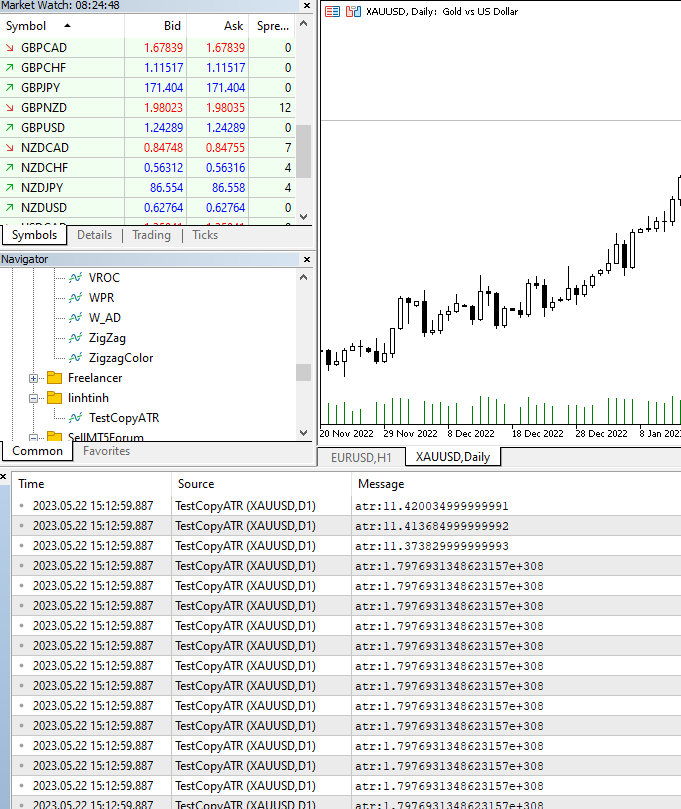# Strange in copybuffer ATR

I found something strange in copybuffer atr indicator. the value return EMPTY_VALUE if shift is far from current. Anyone get this problem?

```//+------------------------------------------------------------------+
//|                                                  TestCopyATR.mq5 |
//|                                                         truongxx |
//+------------------------------------------------------------------+
#property version   "1.00"
#property indicator_chart_window

int handle_atr=0;
//+------------------------------------------------------------------+
//| Custom indicator initialization function                         |
//+------------------------------------------------------------------+
int OnInit()
{
//--- indicator buffers mapping
handle_atr=iATR(_Symbol, PERIOD_CURRENT, 200);
//---
return(INIT_SUCCEEDED);
}
//+------------------------------------------------------------------+
//| Custom indicator iteration function                              |
//+------------------------------------------------------------------+
int OnCalculate(const int rates_total,
const int prev_calculated,
const datetime &time[],
const double &open[],
const double &high[],
const double &low[],
const double &close[],
const long &tick_volume[],
const long &volume[],
{
//---
if(prev_calculated<=0)
{
Print("rates_total:",rates_total);
for(int i=401;i<rates_total;i++)
{
double atr[];
CopyBuffer(handle_atr, 0, i, 1, atr);
Print("atr:",atr);
}

}
//--- return value of prev_calculated for next call
return(rates_total);
}
//+------------------------------------------------------------------+
```Try printing ATR[rates_total-1].
But also you don't need to put CopyBuffer inside a loop it is not good practice. It needs to run only once to get all the values in the buffer. But you will need to search for limit and to_copy to define the lookbacks right.

And I would strongly recommend that you separate for the for loop from the Copybuffer in your project and test both of them on its own. Because like this you are mixing them up until you don't know which is which.

Instead finding out which is which and how they work should be the first thing to do.

Minh Truong Pham: I found something strange in copybuffer atr indicator. the value return EMPTY_VALUE if shift is far from current. Anyone get this problem?

Nothing strange there. To calculate a SMA of length 200, you need at least 200 values. The "a" in ATR means average.

`      for(int i=401;i<rates_total - 199 ;i++)`
Reason: